• Slides: 20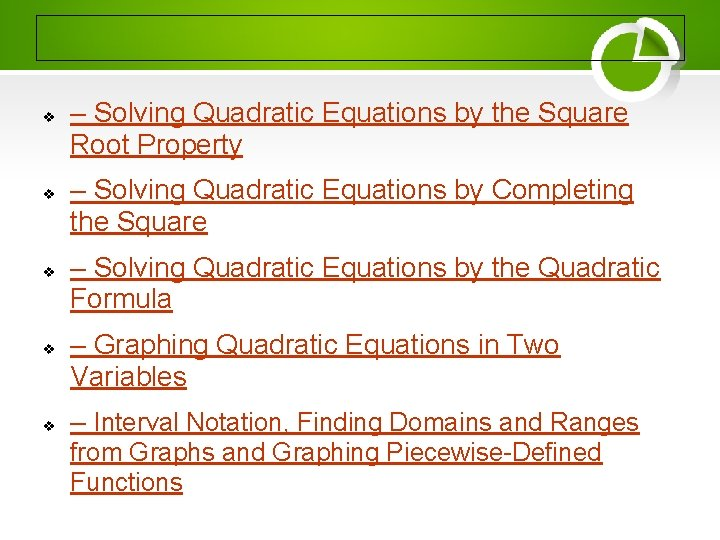v v v – Solving Quadratic Equations by the Square Root Property – Solving Quadratic Equations by Completing the Square – Solving Quadratic Equations by the Quadratic Formula – Graphing Quadratic Equations in Two Variables – Interval Notation, Finding Domains and Ranges from Graphs and Graphing Piecewise-Defined Functions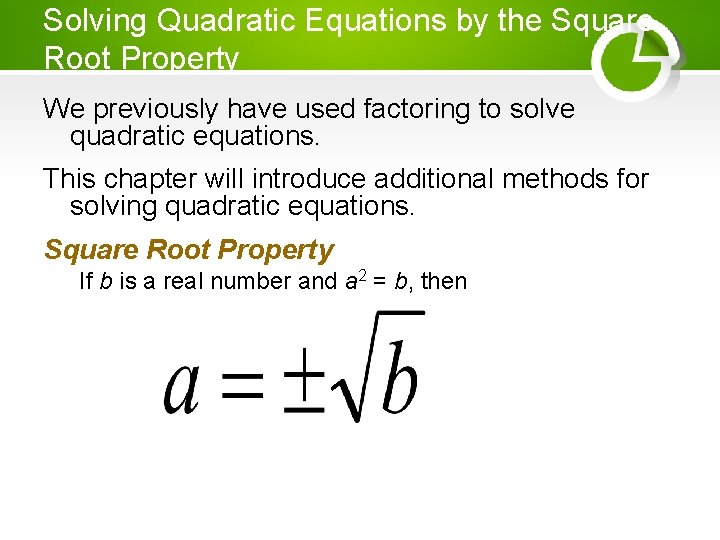Solving Quadratic Equations by the Square Root Property We previously have used factoring to solve quadratic equations. This chapter will introduce additional methods for solving quadratic equations. Square Root Property If b is a real number and a 2 = b, thenSquare Root Property Example Solve (x + 2)2 = 25 x = 2 ± 5 x = 2 + 5 or x = 2 – 5 x = 3 or x = 7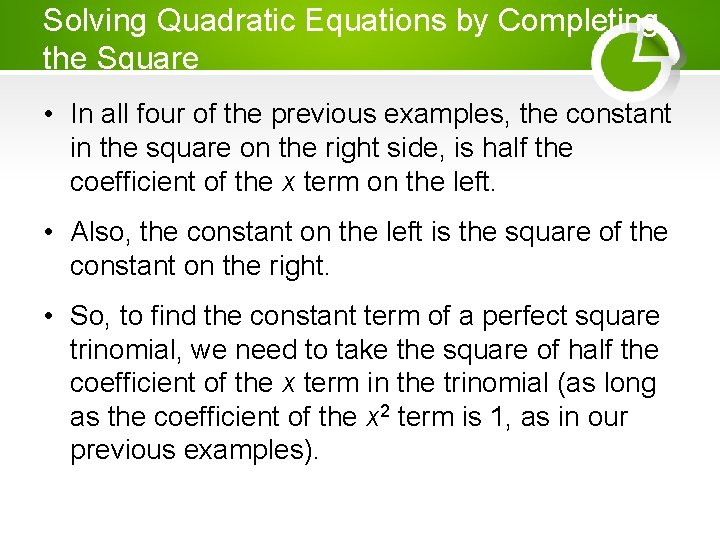Solving Quadratic Equations by Completing the Square • In all four of the previous examples, the constant in the square on the right side, is half the coefficient of the x term on the left. • Also, the constant on the left is the square of the constant on the right. • So, to find the constant term of a perfect square trinomial, we need to take the square of half the coefficient of the x term in the trinomial (as long as the coefficient of the x 2 term is 1, as in our previous examples).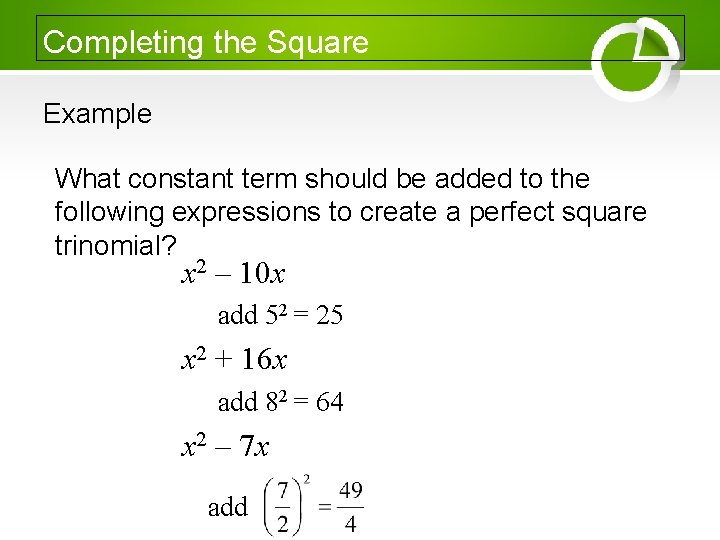Completing the Square Example What constant term should be added to the following expressions to create a perfect square trinomial? x 2 – 10 x add 52 = 25 x 2 + 16 x add 82 = 64 x 2 – 7 x add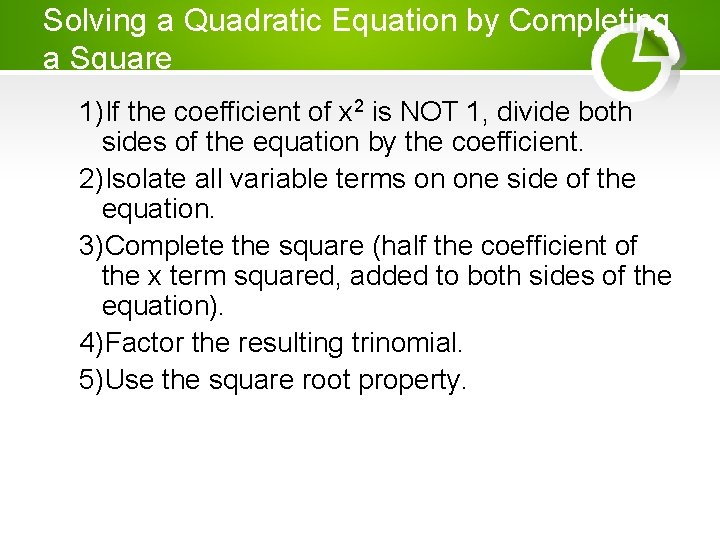Solving a Quadratic Equation by Completing a Square 1)If the coefficient of x 2 is NOT 1, divide both sides of the equation by the coefficient. 2)Isolate all variable terms on one side of the equation. 3)Complete the square (half the coefficient of the x term squared, added to both sides of the equation). 4)Factor the resulting trinomial. 5)Use the square root property.Solving Quadratic Equations by the Quadratic Formula Another technique for solving quadratic equations is to use the quadratic formula. The formula is derived from completing the square of a general quadratic equation.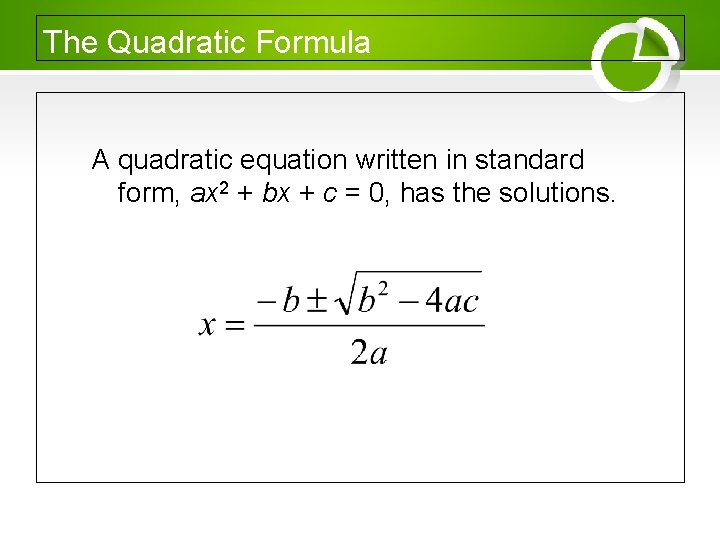The Quadratic Formula A quadratic equation written in standard form, ax 2 + bx + c = 0, has the solutions.The Quadratic Formula Example Solve 11 n 2 – 9 n = 1 by the quadratic formula. 11 n 2 – 9 n – 1 = 0, so a = 11, b = -9, c = -1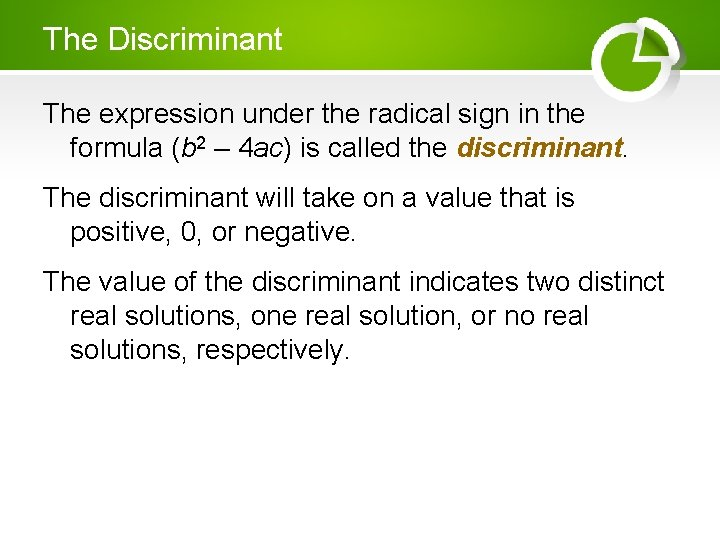The Discriminant The expression under the radical sign in the formula (b 2 – 4 ac) is called the discriminant. The discriminant will take on a value that is positive, 0, or negative. The value of the discriminant indicates two distinct real solutions, one real solution, or no real solutions, respectively.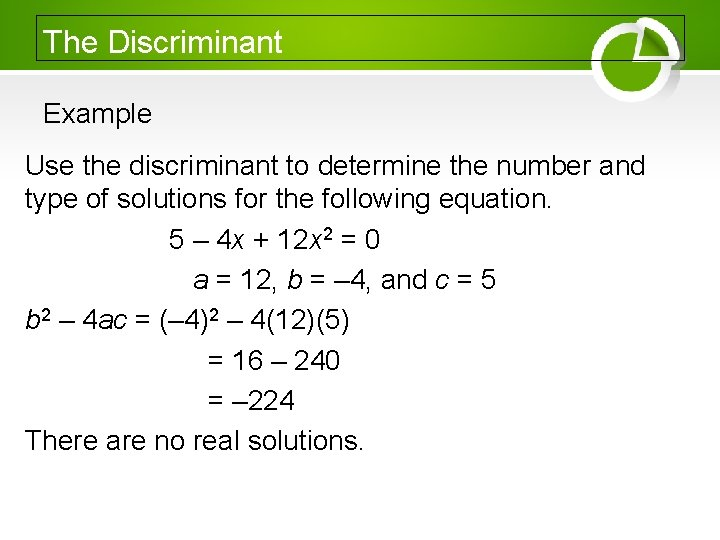The Discriminant Example Use the discriminant to determine the number and type of solutions for the following equation. 5 – 4 x + 12 x 2 = 0 a = 12, b = – 4, and c = 5 b 2 – 4 ac = (– 4)2 – 4(12)(5) = 16 – 240 = – 224 There are no real solutions.Steps in Solving Quadratic Equations 1)If the equation is in the form (ax+b)2 = c, use the square root property to solve. 2)If not solved in step 1, write the equation in standard form. 3)Try to solve by factoring. 4)If you haven’t solved it yet, use the quadratic formula.Solving Equations Solve 12 x = 4 x 2 + 4. 0 = 4 x 2 – 12 x + 4 0 = 4(x 2 – 3 x + 1) Let a = 1, b = -3, c = 1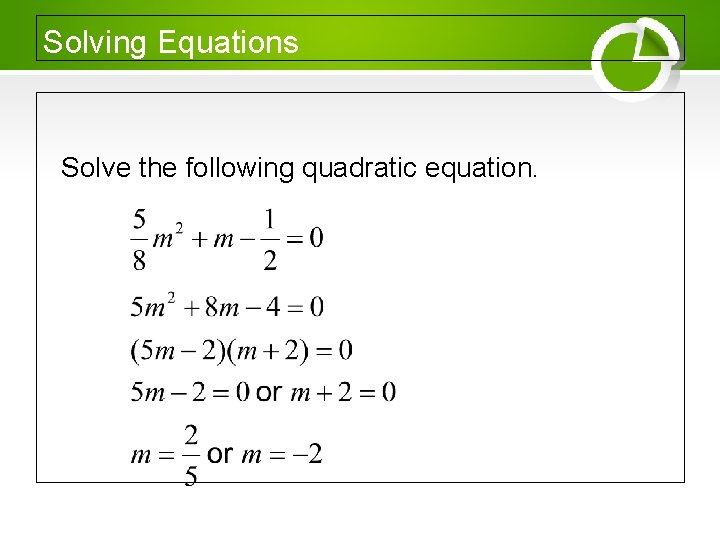Solving Equations Solve the following quadratic equation.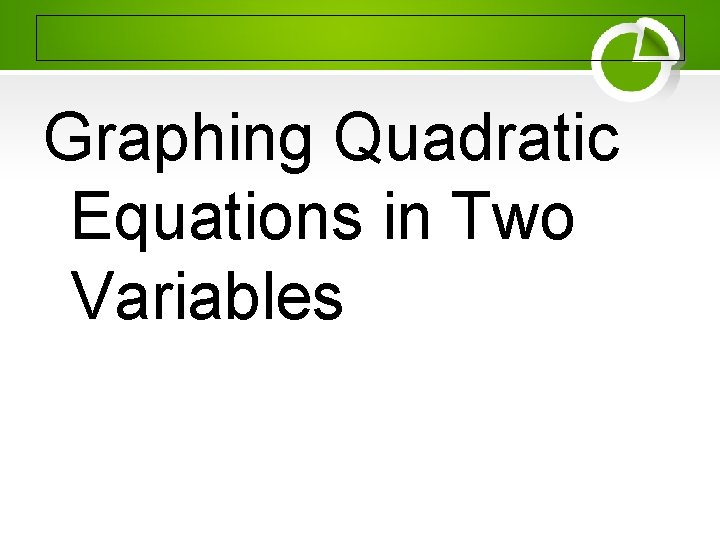Graphing Quadratic Equations in Two Variables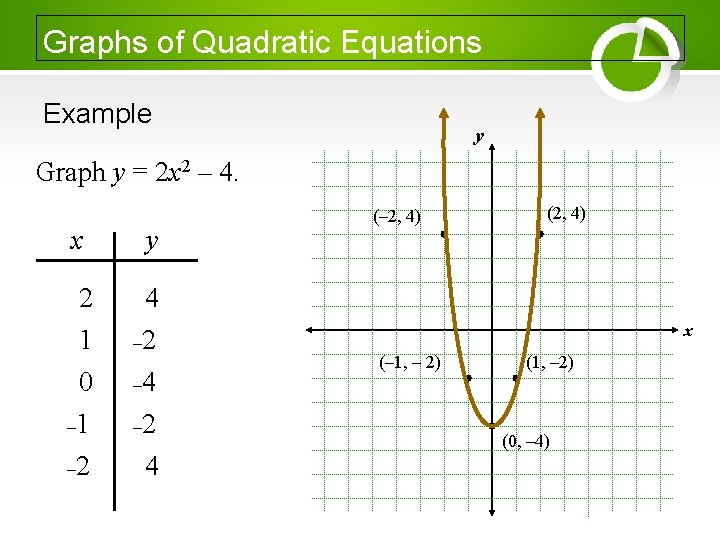Graphs of Quadratic Equations Example y Graph y = 2 x 2 – 4. x y 2 1 0 – 1 – 2 4 – 2 – 4 – 2 4 (– 2, 4) (2, 4) x (– 1, – 2) (0, – 4)Intercepts of the Parabola • Although we can simply plot points, it is helpful to know some information about the parabola we will be graphing prior to finding individual points. • To find x-intercepts of the parabola, let y = 0 and solve for x. • To find y-intercepts of the parabola, let x = 0 and solve for y.Characteristics of the Parabola If the quadratic equation is written in standard form, y = ax 2 + bx + c, 1) the parabola opens up when a > 0 and opens down when a < 0. 2) the x-coordinate of the vertex is To find the corresponding y-coordinate, you substitute the x-coordinate into the equation and evaluate for y. .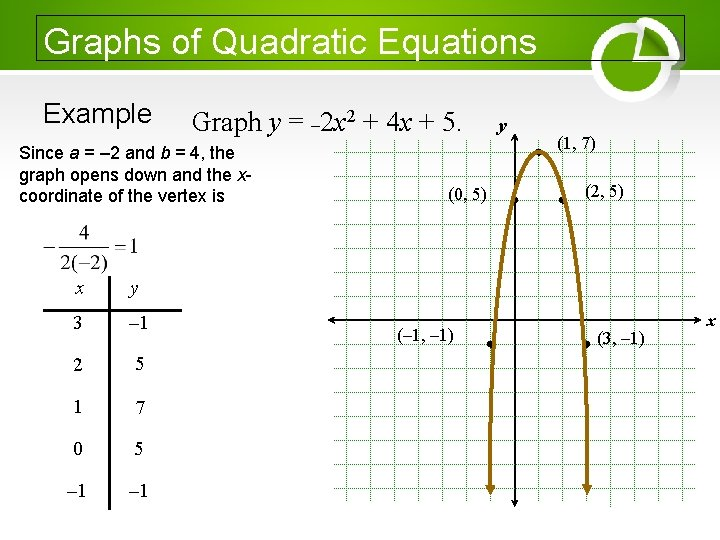Graphs of Quadratic Equations Example Graph y = – 2 x 2 + 4 x + 5. Since a = – 2 and b = 4, the graph opens down and the xcoordinate of the vertex is x y 3 – 1 2 5 1 7 0 5 – 1 (0, 5) (– 1, – 1) y (1, 7) (2, 5) (3, – 1) x